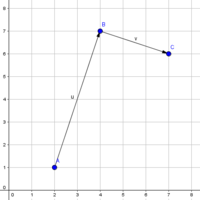# Points on a Lattice

#### rakam

##### New member
I got this question somewhere:

Hanna moves in a lattice where every point can be represented by a pair of integers. She moves from point A to point B and then takes a turn 90 degrees right and starts moving till she reaches the first point on the lattice. Find what's the point she would reach? In essence the problem boils down to finding the first point where the perpendicular to a line will intersect.

I did find one solution on stack overflow but can't understand it. Can someone please explain me the following solution:

Let (dx,dy) = (Bx,By)-(Ax,Ay), the vector from point A to point B.
We can rotate this by 90 degrees to give (dy,-dx).
After hanna turns right at B, she will head out along that rotated vector toward (Bx+dy,By-dx)
Since she is moving in a straight line, her vector from B will follow (t.dy,-t.dx), and will hit another lattice point when both of those components are integers, i.e...
She will hit another lattice point at: (Bx + dy/GCD(|dx|,|dy|), By - dx/GCD(|dx|,|dy|) )

#### Dr.Peterson

##### Elite Member
Please tell us which parts you do understand, and which you don't. In particular, how much do you know about vectors?

I would actually rather guide you through figuring out an answer based on what you already know, than teach you topics you don't know just to understand this person's work. That's a major disadvantage of trying to solve a problem by searching for ready-made answers, rather than thinking for yourself, and is why we don't give ready-made answers.

Also, I would recommend that you start with a drawing of an example. Try this one:#### rakam

##### New member
Thanks Dr. Peterson for the reply.

So I do understand the following:
Let (dx,dy) = (Bx,By)-(Ax,Ay), the vector from point A to point B.
We can rotate this by 90 degrees to give (dy,-dx).
After hanna turns right at B, she will head out along that rotated vector toward (Bx+dy,By-dx)
Since she is moving in a straight line, her vector from B will follow (t.dy,-t.dx), and will hit another lattice point when both of those components are integers, i.e...

The part that I don't understand is:
She will hit another lattice point at: (Bx + dy/GCD(|dx|,|dy|), By - dx/GCD(|dx|,|dy|) )

That is how did we get the 1/GCD(|dx|,|dy|) component.

#### Dr.Peterson

##### Elite Member
Look at my example, where (dx,dy) is u=(2, 6), so (dy,-dx) is (6, -2). Here, GCD(2, 6) = 2, which means we can divide both components by 2 and still get a pair of integers. The resulting vector, v=(3, -1) takes us to the first lattice point we come to, which is B+v = (4,7) + (3,-1) = (7,6), my point C.

This is much like reducing a fraction to lowest terms by dividing terms by the GCD.

#### rakam

##### New member
Ah.. got it
Thank you so much for explanation. Really appreciate your help

#### rahuls

##### New member
Thanks Dr. Peterson. I have one question though.

How did you come at resulting vector v=(3, -1) ?
I came at vector resulting vector v = (3,4) as following:

GCD (6,2 ) => 2
(BX, BY)=> 4 , 7
(dx, dy) => 6 , -2

(Bx + dy/GCD(|dx|,|dy|), By - dx/GCD(|dx|,|dy|) )

(4 - 2/2, 7 - 6/2) ==> (3,4)

#### Dr.Peterson

##### Elite Member
How did you come at resulting vector v=(3, -1) ?
I came at vector resulting vector v = (3,4) as following:

GCD (6,2 ) => 2
(BX, BY)=> 4 , 7
(dx, dy) => 6 , -2

(Bx + dy/GCD(|dx|,|dy|), By - dx/GCD(|dx|,|dy|) )

(4 - 2/2, 7 - 6/2) ==> (3,4)
Divide (6, -2) by 2 and you get the VECTOR v = (3, -1). Right?

I also showed how the POINT C = (4,7) + (3, -1) = (7, 6). That's what the formula is supposed to produce: C, not v.

You also wrongly took (dx,dy) to be my rotated vector (6, -2). But in the formula, (dx,dy) is the unrotated vector u = AB = (2, 6). Putting that in the formula, you get C = (4 + 6/2, 7 - 2/2) = (7, 6) which is C in my picture (again, not v).

#### rahuls

##### New member
Divide (6, -2) by 2 and you get the VECTOR v = (3, -1). Right?

I also showed how the POINT C = (4,7) + (3, -1) = (7, 6). That's what the formula is supposed to produce: C, not v.

You also wrongly took (dx,dy) to be my rotated vector (6, -2). But in the formula, (dx,dy) is the unrotated vector u = AB = (2, 6). Putting that in the formula, you get C = (4 + 6/2, 7 - 2/2) = (7, 6) which is C in my picture (again, not v).
Thank you. Got it.